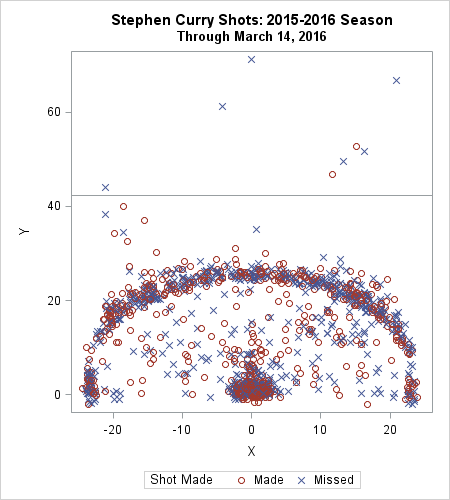Last week Robert Allison showed how to download NBA data into SAS and create graphs such as the location where Stephen Curry took shots in the 2015-16 season to date. The graph at left shows the kind of graphs that Robert created. I've reversed the colors from Robert's version, so that red indicates "good" (a basket was scored) and blue indicates "bad" (a missed shot). The location of the NBA three-point line is evident by the many markers that form an arc in the scatter plot.

When I saw the scatter plot, I knew that I wanted to add some statistical analysis. In particular, I wanted to use SAS to construct a statistical model that estimates the probability that Curry scores from any position on the court.

This article focuses on the results of the analysis. You can download the SAS program that generates the analyses and graphics. Although this article analyzes Stephen Curry, you can modify the SAS program to analyze Kevin Durant, Lebron James, or any other player.

### Probability as a function of distance

The first analysis estimates the probability that Curry makes a basket solely as a function of his distance from the basket. Curry is known for his consistent ability to make three-point shots. A three-point shot in the NBA requires that a player shoot from at least 22 feet away (when near the baseline) or 23.9 feet away (when further up the court).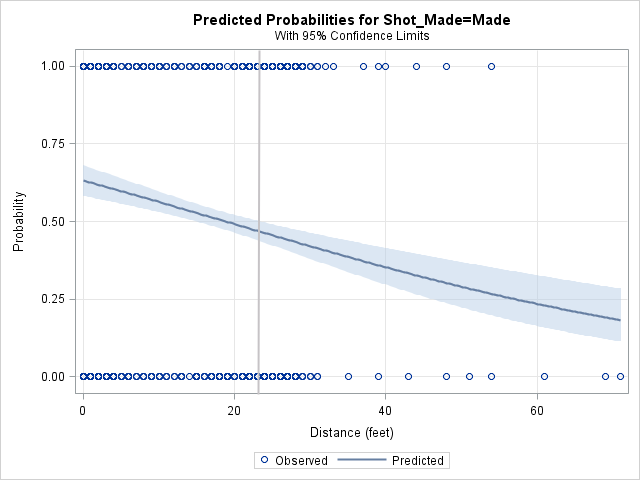You can use logistic regression to model the probability of making a shot as a function of the distance to the basket. The adjacent plot shows the result of a logistic regression analysis in SAS. The model predicts a probability of 0.7 that Curry will make a shot from under the basket, a probability of 0.5 from 20 feet away, and a probability of 0.46 from the three-point arc, indicated by the vertical gray line at 23.9 feet. Recall that a probability of 0.46 is equivalent to predicting that Curry will sink 46% of shots from the three-point arc.

Almost all (98.3%) of Curry's shots were taken from 30 feet or closer, and the shots taken from beyond 30 feet were end-of-quarter "Hail Mary" heaves. Therefore, the remaining analyses restrict to shots that were from 30 feet or closer.

### Probability as a function of angle and distance

The previous analysis only considers the distance from the basket. It ignores position of the shot relative to the basket. In general, the probability of scoring depends on the location from which the shot was launched.

For consistency, let's agree that "right" and "left" means the portion of the court as seen by a fan sitting behind the backboard. This is, of course, opposite of what Curry would see when coming down the court toward the basket. Our "right" is Curry's left.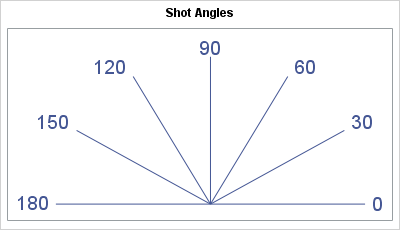One way to model the positional dependence in the model is incorporate the angle relative to the backboard. The diagram shows one way to assign an angle to each position on the court. In the diagram, 90 degrees indicates a perpendicular shot to the basket, such as from the top of the key. An angle of 0 indicates a "baseline shot" from the right side of the court. Similarly, an angle of 180 degrees means a baseline shot from the left side of the court.

The following panel of graphs is the result of a logistic regression analysis that includes the interaction between angle and distance. The vertical lines in some plots indicate the distance to the sideline at particular angles. For 0 and 180 degress, the distance from the basket to the sideline is 25 feet.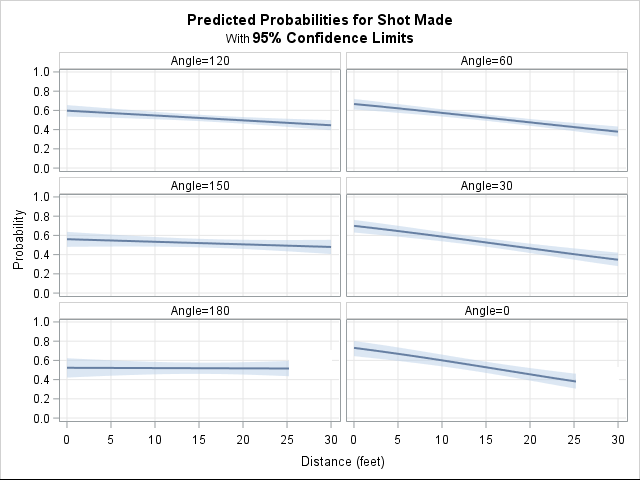The panel of plots show that Curry is most accurate when he shoots from the left side of the court. (The left side corresponds to angles greater than 90 degrees, which are on the left side of the panel.) Remarkably, the model estimates that Curry's probability of making a shot from the left side barely depends on the distance from the basket! He is a solid shooter (probability 0.5, which is 50%) from the left baseline (Angle = 180) and from a slight angle (Angle = 150). The previous scatter plot shows that he shoots many shots from the 120 degree angle. This analysis shows that he is uncannily accurate from 20 and even 30 feet away, although the probability of scoring decreases as the distance increases.

Does Stephen Curry shoot better from his right? #Statistics can tell you! #NBA #Analytics
Click To Tweet

On the right side of the court (angles less than 90 degrees), Curry's probability of making a shot depends more strongly on the distance to the basket. Near the basket, the model predicts a scoring probability of 0.6 or more. However, the probability drops dramatically as the distance increases. On the right side of the court, Curry is less accurate from 20 or more feet than for the same distance on the other side. At three-point range, Curry's probability of making a shot on the right (his left) drops to "only" 0.4. The probability drops off most dramatically when Curry shoots from the baseline (Angle = 0).

### Probability as a function of position

A logistic analysis is a parametric model, which means that the analyst must specify the explanatory variables in the model and also the way that those variables interact with each other. This often leads to simplistic models, such as a linear or quadratic model. A simple model is often not appropriate for modeling the scoring probability as a function of the Cartesian X and Y positions on the court because a simple model cannot capture local spatial variations in the data.

SAS provides several possibilities for nonparametric modeling of data, but let's stick with logistic regression for now. Many SAS regression procedures, including PROC LOGISTIC, support using an EFFECT statement to generate spline effects for a variable. A spline effect expands a variable into spline bases. Spline effects enable you to model complex nonlinear behavior without specifying an explicit form for the nonlinear effects. The following graph visualizes such a model.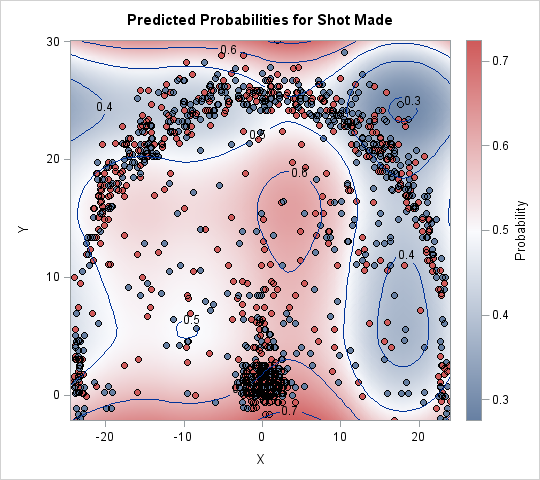The image shows a scatter plot of the location of shots overlaid on a heat map that shows the predicted probability of Curry sinking a basket from various locations on the court. To better show the shot locations, the image has been stretched vertically. As mentioned previously, the location with the highest predicted probability is under the basket. From farther away, the predicted probability varies according to direction: to the left of the basket the probability is about 0.5, whereas a 15-foot jumper in front of the basket has probability 0.6. Notice the relative abundance of blue color (low probability) for shots on the right side. The lowest probability (about 0.3) occurs just beyond the three-point line at about a 60 degree angle, which agrees with the previous analysis. The same distance on the left side of the court is a much lighter shade of whitish-blue that corresponds almost 0.5 probability.

Statisticians will wonder about how well the model fits the data. The Pearson goodness-of-fit test indicates that the spline fit is not great, which is not surprising for a parametric fit to this kind of spatial data. In a follow-up post I will present an alternative nonparametric analysis.

### Conclusions

SAS programmers will appreciate the fact that "effect plots" in this article were generated automatically by PROC LOGISTIC. By using the EFFECT statement and the EFFECTPLOT statement, it is simple to create graphs that visualize the predictions for a logistic regression model.

These graphs show that in general Stephen Curry is a phenomenal shooter who has a high probability of scoring from even a long distance. Logistic regression was used to model the probability that Curry makes a shot from various angles and locations on the court. The analysis indicates that Curry shoots better from his right side, especially from three-point range.

tags: Data Analysis

The post A statistical analysis of Stephen Curry's shooting appeared first on The DO Loop.